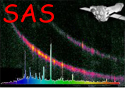XMM-Newton Science Analysis System

ommosaic (ommosaic-2.11) [xmmsas_20211130_0941-20.0.0]

## Initialization (image alignment mode)

```    Read the list of input files on the command-line.

Read the input parameter nsigma
Read the input parameter minnumpixels
Read the input parameter minfraction

Set nFiles to the number of files

Loop through each file (i=1 to nFiles)

Compute the mean  background of the image.
Compute the number of image pixels that have a value >= mean background +
nsigma * sqrt(mean background)
and store this value in the vector numberGoodPixels

End Loop

Create an empty vector, called alignment, that will store details of pairs of
images that can possibly aligned
Loop through each file (i=0 to nFiles-1)
if numberGoodPixels[i] < minnumpixels skip this value of i
Loop through from j=i+1 to nFiles - 1
if numberGoodPixels[j] < minnumpixels skip this value of j
if( the area overlap of the two images divided by the maximum area of the
two images is less than minfraction skip this value of j
Add i, j, and the number of usable pixels to the alignment vector.
End loop
End loop

Sort the alignment vector in order of decreasing overlap area
```

Subsections

XMM-Newton SOC -- 2021-11-30